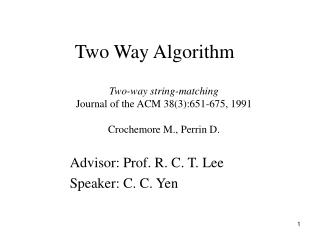DownloadDownload PresentationTwo Way Algorithm

# Two Way Algorithm

Télécharger la présentation## Two Way Algorithm

- - - - - - - - - - - - - - - - - - - - - - - - - - - E N D - - - - - - - - - - - - - - - - - - - - - - - - - - -
##### Presentation Transcript

1. Two Way Algorithm Two-way string-matching Journal of the ACM 38(3):651-675, 1991 Crochemore M., Perrin D. Advisor: Prof. R. C. T. Lee Speaker: C. C. Yen

2. In 2003 ,Rytter proposed a constant space and linear time string matching algorithm • To achieving the good constant space , this algorithm avoids the preprocessing function table of the KMP algorithm • Before introducing this algorithm , we shall define some characteristic of the strings

3. The Property of Maximal Suffix • Consider a string P. Let P = uv where v = MaxSuf(P). The property of the maximal suffix of a string is: If u is non-empty, no suffix of u will be equal to a prefix of v. Example ： Consider a pattern = ababadada. Let P = uv =ababa.dada No suffix of u is equal to a prefix of v.

4. Short Maximal Suffix • If a maximal suffix of a string x satisfies , we say that this maximal suffix of x is a short maximal suffix of x. Example： Consider a string x = abcdda ,dda is a maximal suffix of x and . Hence we say that dda is a short maximal suffix of x

5. Short Prefixes Lemma • Let the decomposition of P = uv, where v is the maximal suffix of P and v is also a short maximal suffix. Suppose that we start to match v with T at position i, a part of v is matched and a mismatch occurs at the j +1-th position on v. Then we can shift P safely by j + 1 positions without missing any occurrence of P in T. i i+j+1 T: mismatch j j P: u v j P: v u

6. j i v’ T: Why do we have to use short maximal suffix? Suppose V’ is very long, then we move pattern which is incorrect. j i v’ P: u v j j+1 T: j i P: u v

7. In the following , we will introduce the basic rule of the Two Way Matching algorithm with short maximal pattern strings The basic rules are given in the next slides.

8. Basic rule of the Two-Way algorithm with short maximal 1. Let the decomposition of P=uv, where v is the maximal suffix of P and v is also a short maximal suffix. • We then find where v appears in T from left to right. Assume the comparison starts at position i. When a mismatch occurs at v[j + 1], we shift v with j + 1 characters and start next comparison at P with T[i + j + 1]. • When the part of v has be found in T, we scan the part of u from right to left. If a mismatch occurs when scanning u, we shift P with Period(P) 4. If we find both the parts of v and u in T, we report an occurrence of P in T. We then shift v with Period(P)